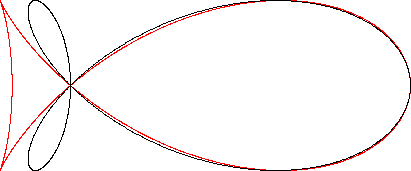Fish Curve
FISH CURVE

Balmoral Software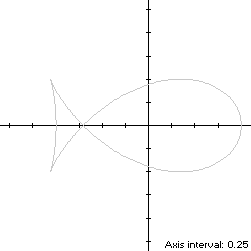The fish curve is an x-symmetric ichthyic closed curve S with parametric equations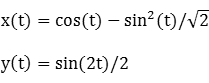for t ∈ T = [0,2π). As t increases from 0, S starts from its right-hand edge at (1,0) and moves through Quadrants I and II, crossing the x-axis at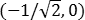when t = π/2 and entering Quadrant III before reversing direction at the fin tip and returning to Quadrant II. It then reverses direction again and continues counterclockwise through Quadrants II, III and IV back to its starting point. The ordinate maxima of S occur at the top of the main lobe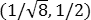when t = π/4 and at the fin tip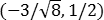when t = 5π/4. Its minimum abscissas also occur at each fin tip, so S is non-convex by the multiple local extrema test. The width x height of its bounding rectangle is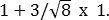### Metrics

The perimeter of the fish curve is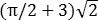and its area is 4/3. Its centroid is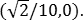### Convex Hull

The convex hull is created by connecting the extreme ordinate and minimum abscissa points with two horizontal line segments of length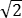and one vertical line segment of length 1. We haveso by (L1) and symmetry, the perimeter of the convex hull is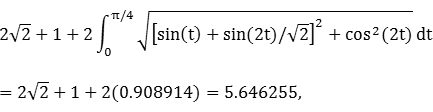which is about 13% shorter than that of the fish curve.

The line segments of the convex hull create a rectangle of area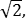as shown in blue in the left diagram below. The centroid abscissa of this rectangle is the average of its vertex abscissas, or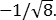By (A1) and symmetry, the remainder of the convex hull has areaBy (C1), the associated centroid abscissa is found by integrating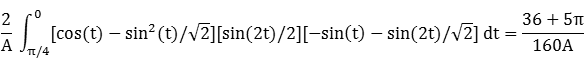Since S is defined parametrically and is traced out in a counterclockwise direction, the usual limits of integration are reversed.

The convex hull component metrics can be summarized as follows:

 Area Centroid abscissa Product Region Rectangle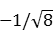-1/2 Remainder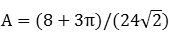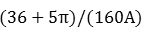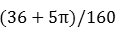Total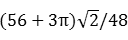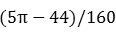The area of the convex hull is 1.927596, about 45% larger than that of the fish curve. The centroid abscissa of the convex hull is the weighted average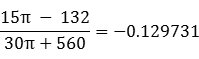### Circumcircle

Using z = 1 in Lemma C, we have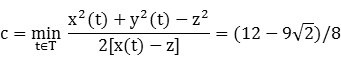and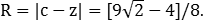For verification, we have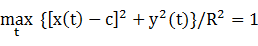### Circumellipse

Lemma E does not produce an ellipse that fully contains S, so we resort to a numerical search to determine the optimal circumellipse:
a = 1.290478

b = 0.640445

c = -0.254244

d = 0

### Incircle (main lobe)

The maximum radius of a circle centered on the x-axis and inscribed in S is its maximum ordinate, so a candidate for the main lobe incircle has radius R = 1/2 and center abscissa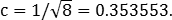The candidate circle must be contained within S, so we require that c - R and c + R both be within the abscissa range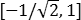of the main lobe of S, which is true. For verification, we have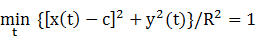### Inellipse (main lobe)

Using z = 1 in Lemma E,has a zero when t* is a complicated expression approximated by 1.083243 radians. The corresponding coordinates are
x* = -0.083459

y* = 0.413881,

from which we have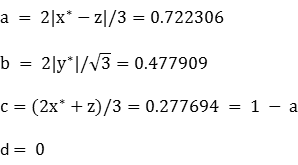For verification,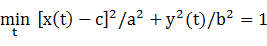### Tail

Inconics can also be found for the tail portion of the fish curve, occurring when t ∈ T = [π/2,3π/2). The left edge of the tail at (-1,0) occurs at t = π when S revisits the x-axis for the third time.

### Incircle (tail)

Using z = -1 in Lemma C, we have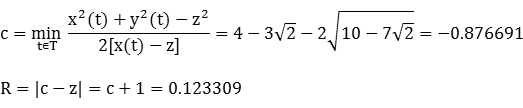### Inellipse (tail)

Using z = -1 in Lemma E,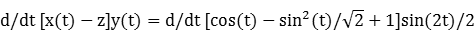has a zero when t* is a complicated expression approximated by 1.749441 radians. The corresponding coordinates are
x* = -0.862475

y* = -0.174868,

from which we have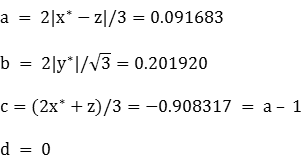For verification, we have### Summary Table

 Perimeter Area Centroid Figure Parameters Incircle (tail)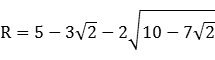0.774771 0.047768 (-0.876691,0) Inellipse (tail) a = 0.091683b = 0.201920 0.955186 0.058159 (-0.908317,0) Incircle (main lobe) R = 1/2 3.141593 0.785398 (0.353553,0) Inellipse (main lobe) a = 0.722306b = 0.477908 3.809772 1.084465 (0.277694,0) Fish curve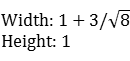6.464082 1.333333 (0.141421,0) Convex hull 5.646255 1.927596 (-0.129731,0) Circumellipse a = 1.290478b = 0.640445 6.239295 2.596464 (-0.254244,0) Circumcircle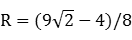6.854894 3.739311 (-0.090990,0)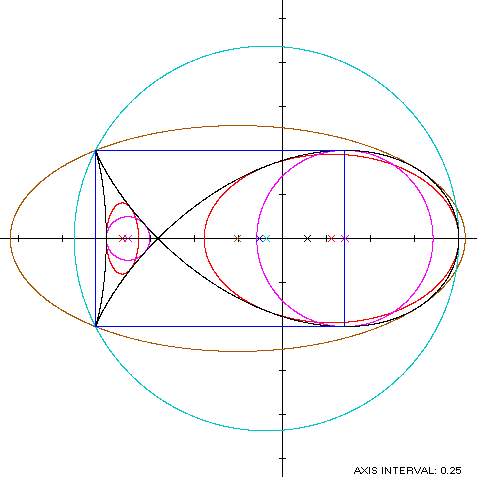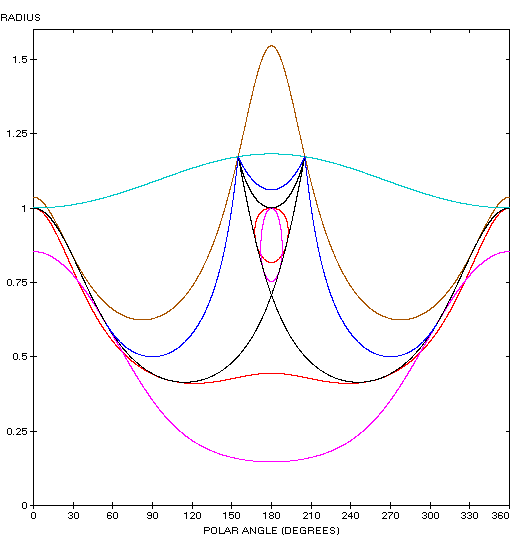The fish curve (red) is similarly shaped to the trefoil: